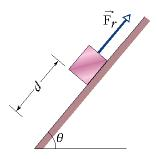# Work Done by the Gravitational Force

I can not figure out what I am doing wrong for this problem. Right now I have Wt = 60*.3*cos(50) = 12 J. If that is taken away, then it should be a 12 J difference right?

In Figure 7-33, a block of ice slides down a frictionless ramp at angle = 50°, while an ice worker pulls up the ramp (via a rope) with a force of magnitude Fr = 60 N. As the block slides through distance d = 0.30 m along the ramp, its kinetic energy increases by 80 J. How much greater would its kinetic energy have been if the rope had not been attached to the block?Work = Force * distance

Remember, you only need to multiply stuff by the cos (angle) or sin (angle) to get that component of force so it matches up with movement.

In this case, the 60 N applied in the opposite direction as the movement...so you don't need to find any of its components.

Simply, you would have a force of 60 N, and a distance of .30 m. With this info, you can easily find the energy.

Doc Al
Mentor
AtlBraves said:
I can not figure out what I am doing wrong for this problem. Right now I have Wt = 60*.3*cos(50) = 12 J. If that is taken away, then it should be a 12 J difference right?
The force and the displacement are both parallel to the surface of the ramp.

I made that problem much harder than I should have. I understand now. Thanks for the help.